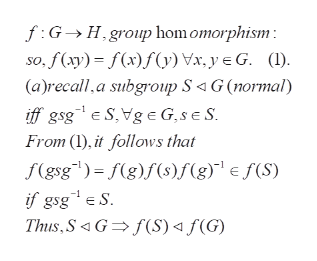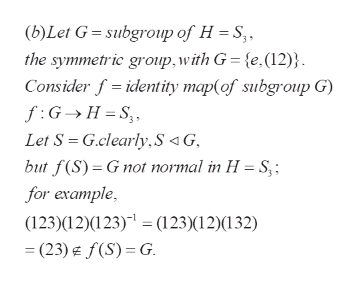# 4*. Let f GH be a group homomorphism. Prove:(a) If SG then f(S) 4 f(G)(b) Show by example that S aG need not imply f(S)(c) If T H then f1(T) G.H

Questionhelp_outlineImage Transcriptionclose4*. Let f G H be a group homomorphism. Prove: (a) If S G then f(S) 4 f(G) (b) Show by example that S aG need not imply f(S) (c) If T H then f1(T) G. H fullscreen
check_circle

Step 1

To prove the stated properties of group homomorphisms

Step 2

proof of (a): the image f(S) is normal in the image f(G) . (note that f(G) need not be H)help_outlineImage Transcriptionclosef:GH.group homomorphism so, f(y) f(x)(y) Vx, y G. () (a)recall.a subgroup S< G (normal) if gsg SVg G,s E S. From () it follows that f(gsg) f(g)f(s)f (g)" e f(\$) if gsgS Thus,S Gf(S) 4 f(G) fullscreen
Step 3

(b) example for S, G , H and f, where S is norm...help_outlineImage Transcriptionclose(b)Let G subgroup of H S the symmetric group,with G {e,(12)} Consider f identity map(of subgroup G) f GH S, Let S G.clearly,S 4 G but f(S) G not normal in H S for example (123)(12)123)1 (123)(12)(l32) f(S) G. = (23) fullscreen

### Want to see the full answer?

See Solution

#### Want to see this answer and more?

Solutions are written by subject experts who are available 24/7. Questions are typically answered within 1 hour.*

See Solution
*Response times may vary by subject and question.
Tagged in

### Math